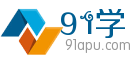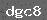面向未来，活在当下！ 收藏夹

 首页 > 数学 > 高考题 > 2022 > 2022年上海春

# 2022年高考数学上海春5

 （4分）设函数$f(x)=x^{3}$的反函数为$f^{-1}(x)$，则$f^{-1}(27)=$　3　．分析：直接利用反函数的定义求出函数的关系式，进一步求出函数的值．解：函数$f(x)=x^{3}$的反函数为$f^{-1}(x)$，整理得${f}^{-1}(x)=\sqrt{x}$；所以$f^{-1}(27)=3$．故答案为：3．点评：本题考查的知识要点：反函数的定义和性质，主要考查学生的运算能力和数学思维能力，属于基础题． 全网搜索"2022年高考数学上海春5"相关
 相关知识
 无相关信息
 发表笔记 共有条笔记
 验证码:学习笔记（共有 0 条）

（微信中可直接长按微信打赏二维码。）
 微信 支付宝